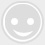# 【51系列教程第六讲】 无法逃避的宿命--定时器

-回复 -浏览51单片机设计了2个8位的特殊功能寄存器，用来控制T/C的工作状态；这两个特殊功能寄存器分别是

TMOD和TCON，都在特殊功能寄存器区，贴两个定时器中断寄存器，有前面中断的基础。应该是比较好理解的了，工作模式控制寄存器TMOD

#include <reg51.h>
sbit LED2 = P1^0;
void main()
{
TMOD=0x01;//设置定时器0为工作方式1
TH0=0xfc;//设置初值，计划1ms定时器溢出中断(我用的是11.0592Mhz晶振)
TL0=0X66;
EA=1;//开总中断
ET0=1;//开定时器0中断
TR0=1;//启动定时器0
while(1)
{}
}
void time0() interrupt 1
{
LED2 = ~LED2;
/*重新配置计数器*/
TF0 = 0;
TH0 = 0xfc;
TL0 = 0x66;
}

#include <reg51.h>
sbit LED = P1^0;
unsigned int Count=0; //定义一个无符号整型变量
void main()
{
TMOD=0x01;//设置定时器0为工作方式1
TH0=0xfc; //定时1ms
TL0=0X66;
EA=1;//开总中断
ET0=1;//开定时器0中断
TR0=1;//启动定时器0
while(1)
{
if(Count ==500) //如果有500次自加，也就是500ms
{
Count = 0;
LED = ~LED; //LED取反
}
}
}
void time0() interrupt 1
{
Count ++; //变量每1ms自加1
TF0 = 0;
TH0 = 0xfc;
TL0 = 0x66;
}